Computational & Technology Resources
an online resource for computational,
engineering & technology publications
Civil-Comp Proceedings
ISSN 1759-3433
CCP: 73
PROCEEDINGS OF THE EIGHTH INTERNATIONAL CONFERENCE ON CIVIL AND STRUCTURAL ENGINEERING COMPUTING
Edited by: B.H.V. Topping
Paper 33

Impact Envelope Formula of Simple Beams due to High Speed Trains

J.D. Yau+ and Y.B. Yang*

+Department of Architecture and Building Technology, Tamkang University, Taipei, Taiwan
*Department of Civil Engineering, National Taiwan University, Taipei, Taiwan

Full Bibliographic Reference for this paper
J.D. Yau, Y.B. Yang, "Impact Envelope Formula of Simple Beams due to High Speed Trains", in B.H.V. Topping, (Editor), "Proceedings of the Eighth International Conference on Civil and Structural Engineering Computing", Civil-Comp Press, Stirlingshire, UK, Paper 33, 2001. doi:10.4203/ccp.73.33
Keywords: impact formula, dynamics, high speed trains, resonance.

Summary
The impact response of bridge structures subjected to moving loads at high speeds is a problem of great concern in the design of high-speed railway bridges. Consider a series of loads moving over the simple beam as shown in Figure 33.1. By neglecting the effect of damping, a closed form solution for the resonant response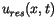of the simple beam can be written as: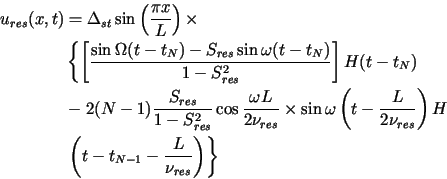(33.1)

where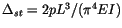is the maximum static deflection of the simple beam,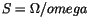is the nondimensional speed parameter,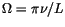is the driving frequency,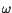is the fundamental natural frequency, and the subscript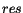means resonance. The term containing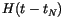in equation (33.1) represents the dynamic response of the beam induced by the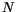th (i.e., the last) moving load, and the term containing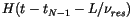indicates the residual response induced by the loads that have passed the beam. As can be seen, under the condition of resonance, larger response will be induced on the beam, as there are more loads passing the beam.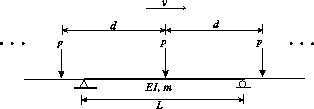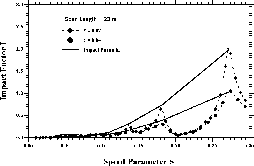On the other hand, whenever the following condition is met, that is,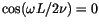, the dynamic response for cancellation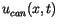in equation (33.1) becomes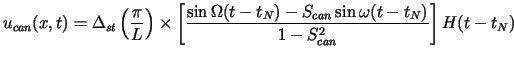(33.2)

where the subscript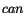means resonance. This implies that the excitation effects of all the previous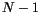moving loads sum to zero. Such a condition has been referred to as the condition of cancellation. As can be seen, whenever the condition of cancellation is met, the response of the beam is determined solely by theth moving load.

In engineering practice, the damping effect of structures should be included. In this study, the damping ratio of simple beams is assumed to be small, i.e., with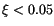, and the operation speed range is assumed to be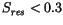) for moving vehicles, which implies that terms involving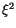and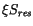, can be neglected. Under such a circumstance, the resonant response of the simple beam subjected to a sequence of moving loads can be obtained as: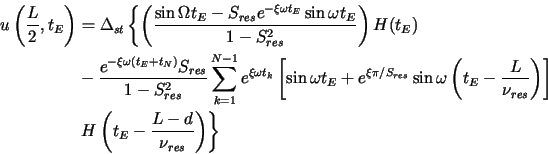(33.3)

where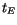indicates the time when theth moving load starts to act on the beam, i.e.,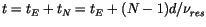. Further, by the approximation in expansion for the exponential function,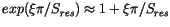for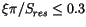, the deflection impact formulas for the simple beam subjected to moving train loads can be derived as: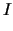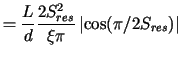for resonance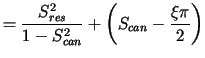for resonance          + cancellation (33.4)

For different damping ratios, the envelope impact formula of equation (33.4) has been plotted in Figure 33.2. As can be seen, for the case of light damping, the envelope impact formula has a trend in good consistency with the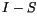relations for the entire range of speed parameters considered.

purchase the full-text of this paper (price £20)